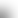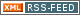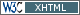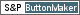www.LinuxHowtos.org howtos, tips&tricks and tutorials for linuxfrom small one page howto to huge articles all in one place

search text in:

Poll
Which linux distribution do you use?

poll results

using iotop to find disk usage hogs

using iotop to find disk usage hogs

words:

887

views:

165658

userrating:

average rating: 1.8 (88 votes) (1=very good 6=terrible)

May 25th. 2007:
Words

486

Views

248876

Workaround and fixes for the current Core Dump Handling vulnerability affected kernels

Workaround and fixes for the current Core Dump Handling vulnerability affected kernels

words:

161

views:

133642

userrating:

average rating: 1.3 (28 votes) (1=very good 6=terrible)

April, 26th. 2006:

You are here: Programming->C/C++

# creating a gauge part 2

Ok, we got our main function and some dummy functions to get some cpu and bandwidth values.

Our graphics will be created using the libgd toolkit, which makes dealing with the images quite easy.

To be able to use it, include gd.h into your program.
`#include <gd.h>`

## MakeImage

```void MakeImage(int cpu, int bandwidth)
{
FILE *fh=fopen("input.png","r");
FILE *out=fopen("output.jpg","w");
if (fh & out)
{
im=gdImageCreateFromPng(fh);
fclose(fh);
drawpointer(im,cpu,1);
drawpointer(im,bandwidth,2);
gdImageJpeg(im, out,-1);
fclose(out);
gdImageDestroy(im);
}
}```
This creates an image from a png file (input.png), draws 2 pointers into the image and writes a jpg file.
The actual drawing will be done in drawpointer.

## drawpointer

We have the cpu and bandwith values stored as an integer from 0-100.
These values need to be converted into angle for our pointer.
```angle=(58.0+(246.0*percent)/100.0);
angle=(angle/180.0)*3.1415;  ```
The first line converts the percent value int an angle between 58 and 246. All functions that will follow need the angle not in °, they need it in a 0-2*pi range.
The second line does the converting.
Optimizing the above lines is left for the reader.
The pointer will be drawn as a solid bright red polygon surrounded by a darker red line.

To get the coordinates for the polygon, the following lines are needed:
```points.x=-4.0*cos(angle)+XOffset;
points.y=-4.0*sin(angle)+YOffset;
points.x=4.0*cos(angle)+XOffset;
points.y=4.0*sin(angle)+YOffset;
gdImageFilledPolygon(im, points, 4, red_bright);
gdImagePolygon(im, points, 4, red_dark);```
This will create the needed coordinates and will draw them to the image. XOffset and YOffset will be calculated depending on the given parameters (1 or 2 as the third argument).
The complete function drawpointer:
```void drawpointer(gdImagePtr im, int percent, int factor)
{
double angle=0;
gdPoint points;
double XOffset=66.0+132*(factor-1);
double YOffset=61.0;
int red_bright;
int red_dark;
int white;
angle=(58.0+(246.0*percent)/100.0);
angle=(angle/180.0)*3.1415;
red_bright = gdImageColorAllocate(im, 250, 50, 0);
red_dark = gdImageColorAllocate(im, 150, 20, 0);
white = gdImageColorAllocate(im, 255, 255, 255);
points.x=-4.0*cos(angle)+XOffset;
points.y=-4.0*sin(angle)+YOffset;
points.x=4.0*cos(angle)+XOffset;
points.y=4.0*sin(angle)+YOffset;
gdImageFilledPolygon(im, points, 4, red_bright);
gdImagePolygon(im, points, 4, red_dark);
}```
The last remaining step is to get the actual cpu and bandwidth information. This will be the last part of this tutorial.
current rating: average rating: 1.0 (12 votes) (1=very good 6=terrible)
Very good (1) Good (2) ok (3) average (4) bad (5) terrible (6)

back||- Powered by- Running on-
Copyright 2004-2020 Sascha Nitsch Unternehmensberatung GmbH::- Level Triple-A Conformance to Web Content Accessibility Guidelines 1.0 -
- Copyright and legal notices -